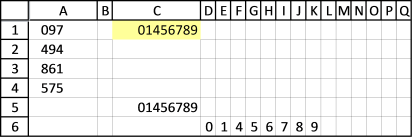Author: Oscar Cronquist Article last updated on August 15, 2017

Find and Sort
I have question, in range A1,A2,A3,A4 contain 097 494 861 575.
What is the formula in excel if the result that i want is 01456789I use two formulas, one array formula in cell range D6:K6 and a regular formula in cell C5.

Formula in cell C5:

=CONCATENATE(D6,E6,F6,G6,H6,I6,J6,K6,L6,M6,N6,O6,P6,Q6)

This formula concatenates values in cell range D6:Q6.

Array formula in cell D6:

=IFERROR(SMALL(IF(COUNTIF(\$C\$6:C6, MID(\$A\$1:\$A\$4,{1,2,3}, 1)*1), "", MID(\$A\$1:\$A\$4, {1,2,3}, 1)*1), 1), "")

Enter array formula in cell D6 and press and hold CTRL + SHIFT simultaneously and then press Enter. Check your formula in the formula bar, it should begin and end with a curly bracket. Like this:

{=IFERROR(SMALL(IF(COUNTIF(\$C\$6:C6, MID(\$A\$1:\$A\$4,{1,2,3}, 1)*1), "", MID(\$A\$1:\$A\$4, {1,2,3}, 1)*1), 1), "")}

Copy cell D6 and paste to E6:Q6.

### Explaining the array formula

• The formula splits all characters into single digit numbers.
• Checks that only unique values are returned.
• Sorts the array from 0 to 9 and returns values in a cell each.

Find and sort numbers.xlsx

### Functions in this post

IF(logical_test, [value_if_true], [value_if_false])
Checks whether a condition is met, and returns one value if TRUE, and another value if FALSE

COUNTIF(range,criteria)Counts the number of cells within a range that meet the given condition

SMALL(array,k)
Returns the k-th smallest number in this data set.

IFERROR(value, value_if_error)
If the value argument returns an error, the value_if_error argument is used. If the value argument does NOT return an error, the IFERROR function returns the value argument.

MID(text, start_num, num_chars)
Returns the characters from the middle of a text string, given starting position and length